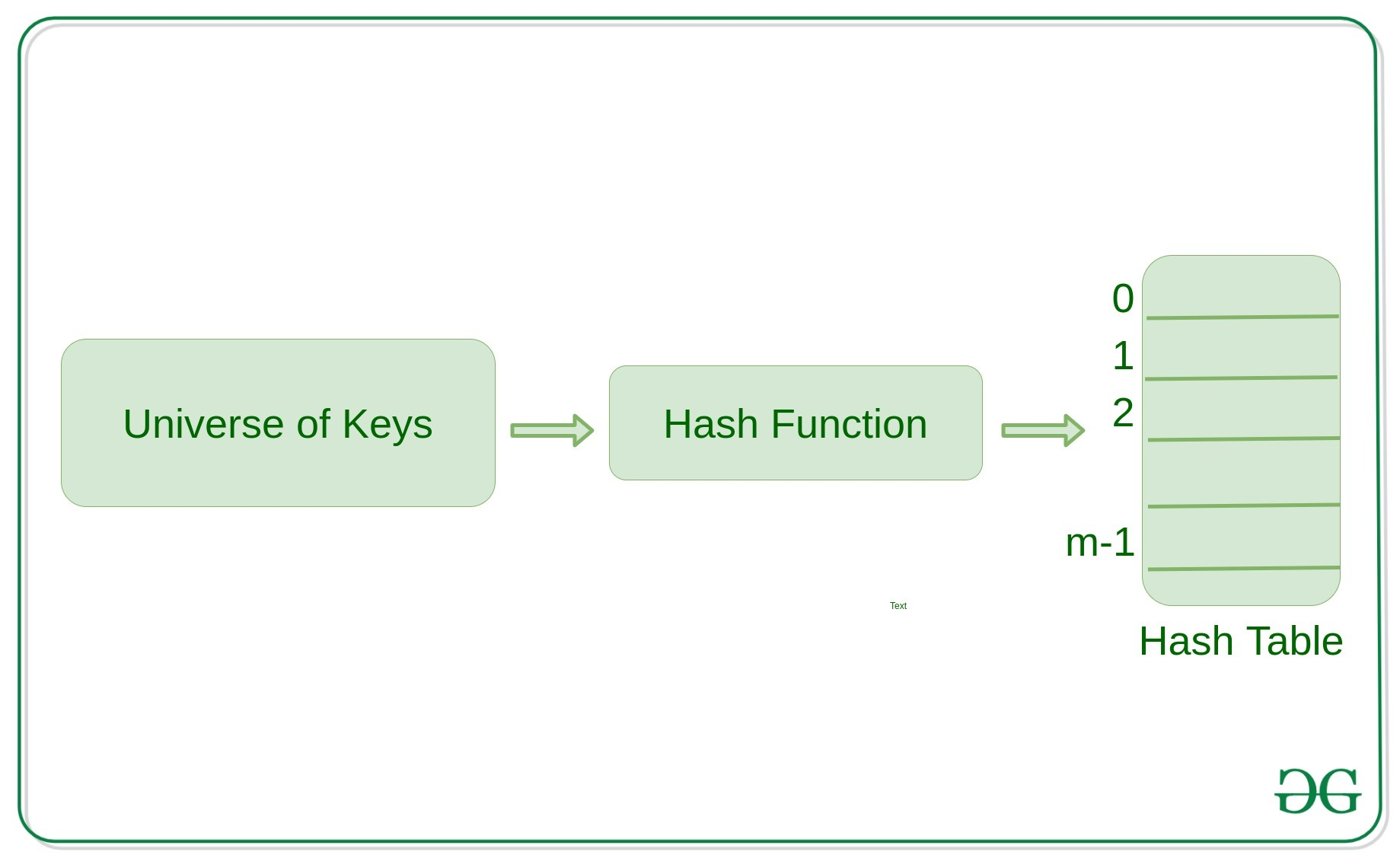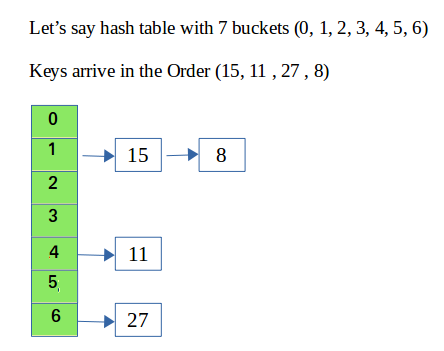# Implementation of Hashing with Chaining in Python

Hashing is a data structure that is used to store a large amount of data, which can be accessed in `O(1)` time by operations such as search, insert and delete. Various Applications of Hashing are:

• Indexing in database
• Cryptography
• Symbol Tables in Compiler/Interpreter
• Dictionaries, caches, etc.

### Concept of Hashing, Hash Table and Hash Function

Hashing is an important Data Structure which is designed to use a special function called the Hash function which is used to map a given value with a particular key for faster access of elements. The efficiency of mapping depends of the efficiency of the hash function used.

Example:

`h(large_value) = large_value % m`

Here, `h()` is the required hash function and ‘m’ is the size of the hash table. For large values, hash functions produce value in a given range.How Hash Function Works?

• It should always map large keys to small keys.
• It should always generate values between 0 to m-1 where m is the size of the hash table.
• It should uniformly distribute large keys into hash table slots.
• ### Collision Handling

If we know the keys beforehand, then we have can have perfect hashing. In perfect hashing, we do not have any collisions. However, If we do not know the keys, then we can use the following methods to avoid collisions:

• Chaining

#### Chaining

While hashing, the hashing function may lead to a collision that is two or more keys are mapped to the same value. Chain hashing avoids collision. The idea is to make each cell of hash table point to a linked list of records that have same hash function value.Note: In Linear Probing, whenever a collision occurs, we probe to the next empty slot. While in Quadratic Probing, whenever a collision occurs, we probe for `i^2th` slot in the ith iteration and we keep probing until an empty slot in the hashtable is found.

### Performance of Hashing

The performance of hashing is evaluated on the basis that each key is equally likely to be hashed for any slot of the hash table.

```m = Length of Hash Table
n = Total keys to be inserted in the hash table

Expected time to search = O(1 +lf )
Expected time to insert/delete = O(1 + lf)

The time complexity of search insert and delete is
O(1) if  lf is O(1)
```

Python Implementation of Hashing

 `# Function to display hashtable ` `def` `display_hash(hashTable): ` `     `  `    ``for` `i ``in` `range``(``len``(hashTable)): ` `        ``print``(i, end ``=` `" "``) ` `         `  `        ``for` `j ``in` `hashTable[i]: ` `            ``print``(``"-->"``, end ``=` `" "``) ` `            ``print``(j, end ``=` `" "``) ` `             `  `        ``print``() ` ` `  `# Creating Hashtable as  ` `# a nested list. ` `HashTable ``=` `[[] ``for` `_ ``in` `range``(``10``)] ` ` `  `# Hashing Function to return  ` `# key for every value. ` `def` `Hashing(keyvalue): ` `    ``return` `keyvalue ``%` `len``(HashTable) ` ` `  ` `  `# Insert Function to add ` `# values to the hash table ` `def` `insert(Hashtable, keyvalue, value): ` `     `  `    ``hash_key ``=` `Hashing(keyvalue) ` `    ``Hashtable[hash_key].append(value) ` ` `  `# Driver Code ` `insert(HashTable, ``10``, ``'Allahabad'``) ` `insert(HashTable, ``25``, ``'Mumbai'``) ` `insert(HashTable, ``20``, ``'Mathura'``) ` `insert(HashTable, ``9``, ``'Delhi'``) ` `insert(HashTable, ``21``, ``'Punjab'``) ` `insert(HashTable, ``21``, ``'Noida'``) ` ` `  `display_hash (HashTable) `

Output:

```0 --> Allahabad --> Mathura
1 --> Punjab --> Noida
2
3
4
5 --> Mumbai
6
7
8
9 --> Delhi ```

Attention geek! Strengthen your foundations with the Python Programming Foundation Course and learn the basics.

To begin with, your interview preparations Enhance your Data Structures concepts with the Python DS Course.

My Personal Notes arrow_drop_upCheck out this Author's contributed articles.

If you like GeeksforGeeks and would like to contribute, you can also write an article using contribute.geeksforgeeks.org or mail your article to contribute@geeksforgeeks.org. See your article appearing on the GeeksforGeeks main page and help other Geeks.

Please Improve this article if you find anything incorrect by clicking on the "Improve Article" button below.

Article Tags :
Practice Tags :

4

Please write to us at contribute@geeksforgeeks.org to report any issue with the above content.